# CONDUCTANCE AND ITS MEASUREMENT

### Conductance of Solutions

Electrical conduction is a property of ionic solutions. From a macroscopic point of view, ionic conduction of solutions is similar to electron or hole conduction through solid objects. In the latter cases electrones are moving without ion cores, while in the former, charges are moving as ions. Although water itself is a very poor conductor of electricity, the presence of ionic species in solution increases the conductance considerably. The conductance of such electrolytic solutions depends on the concentration of the ions and also on the nature of the ions present (through their charges and mobilities), and conductance behavior as a function of concentration is different for strong and weak electrolytes. If the charge dQ is transferred during time dt, the current:Experimental measurement of conductance

(i) The conductance of a solution is reciprocal of the resistance, therefore, the experimental determination of the conductance of a solution involves the measurement of its resistance.

(ii) Calculation of conductivity: We have seen that conductivity (k)is reciprocal of resistivity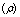, i.e.,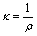and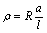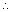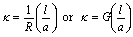where G is the conductance of the cell, l is the distance of separation of two electrodes having cross section area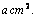The quantity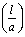is called cell constant and is expressed in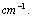Knowing the value of cell constant and conductance of the solution, the specific conductance can be calculated as,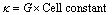i.e.,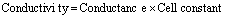I = dQ/dt                                      (1)

is passing through the sample. Electrolyte solutions obey Ohm’s law just as metallic conductors do. The current I passing through a given body of solution is proportional to the applied potential difference V. The resistance R of the body of solution in ohms (W) is given by:

R = V/I                                      (2)

where the potential difference is expressed in volts and the current in amperes. The conductance, defined as the reciprocal of the resistance, of a homogeneous body of uniform cross section is proportional to the cross-sectional area A and inversely proportional to the length l, as shown in the Figure on the right:

G = 1/R = kA/l                                  (3)

where k is the conductivity with units W-1m-1:

k = l/AR = C/R                                  (4)

(By international agreement, the reciprocal ohm, W-1is now called a siemens1 S = 1 W-1). One can think of the conductivity as the conductance of a cube of material, 1 m on each edge. Since it is difficult to build a cell with well defined geometrical parameters A and l, any cell should be calibrated with a solution of exactly known specific conductance. From Eq.(5) we can determine the cell constant C :

C = l/A                                  (5)

The cell constant, C, with units m-1 , can be also determined by measuring the resistance of a cell filled with a solution of known conductivity, which is invariably KCl (usually 0.0200 M). Once the cell constant C has been determined, conductivity of any solution can be calculated from experimental resistances by using Eq. (4). The conductivity, k, increases with concentration, c, and mobilities, m+ and m, of ions:

k = 1000acF(n+z+m+ + n|z|m) = 1000ancF(m+ + m)                            (6)

where F = 96485.3 C/mol – is the Faraday constant and the concentration is expressed in equivalents per mole units, anc, reflecting that: a) a salt An+Bn upon dissolving forms Az+ and Bz ions, i.e., total of n = n+z+ + n|z| ions; b) ionization happens incompletely but only as a fraction. Thus, a more fundamental unit of electrolytic conductance, the equivalent conductance L, can be defined as the value of k contributed by one equivalent of ions contained in 1 L of solvent:

L = k/1000nc = aF(m+ + m)                          (7)

The units of concentration, c, are equiv/L and the units of L are m2equiv-1W-1 and the mobility has the units m2s-1V-1. Note, that in the case of simple one-one electrolytes A+B, there is no distinction between equivalents and moles and the conductivity is the same as the molar conductance Lm.

Strong Electrolytes.

While the conductivity, k, of a solution increases with concentration, the equivalent conductance, L, decreases as the concentration increases. Onsager showed theoretically for strong electrolytes in dilute solution that the effect of ionic attraction reduces the equivalent conductance:

L = L0 (1 – bc1/2)                          (8)

L0 is the equivalent conductance at infinite dilution, which can be obtained by measuring conductance as a function of concentration. Thus, a plot of L versus c1/2 should yield a straight line (when c is less than 0.1 M), the intercept being L0. The L0 values are found to be additive. This suggests that these values are the sums of the independnet cotributions of each of the ions present in the electrolyte. Thus, for example,

L0(NaCl) = l0(Na+) + l0(Cl)                          (9)

The Table of conductivities of some strong electrolytes is presented below

 Equivalent conductances (m2 equiv-1 W-1) of some strong electrolytes at 25oC Electrolyte L0 dL0/dT Electrolyte L0 dL0/dT HCl 0.042616 6.4 × 10-4 CaCl2 0.013584 LiCl 0.011503 Ca(NO3)2 0.013094 NaCl 0.012645 BaCl2 0.013998 KCl 0.014986 2.8 × 10-4 NaNO3 0.012155 KBr 0.015190 KNO3 0.014496 KO2CCH3 0.01114 2.1 × 10-4 NaO2CCH3 0.009100

### Weak Electrolytes.

For a weakly ionized substance, L varies much more markedly with concentration because the degree of ionization, a, varies strongly with concentration. The equivalent conductance, however, must approach a constant finite value at infinite dilution, L0, which again corresponds to the sum of the limiting ionic conductances. It is usually impractical to determine this limiting value from extrapolation of L values obtained with the weak electrolyte itself, since to obtain an approach to complete ionization the concentration must be made too small for effective measurement of conductance. However, L0 for a weak electrolyte can be deduced from Lvalues obtained for strong electrolytes similar to Eq. (9). As an example, let us consider acetic acid (CH3COOH, denoted as HAc) as a typical weak electrolyte. Its equivalent conductance can be calculated using:

L0(HAc) = L0(HCl) + L0(NaAc) – L0(NaCl)                                  (10)

equivalent conductances of HCl, NaAc and NaCl.

For sufficiently weak electrolytes, the ionic concentration is small and the effect of ion attraction on the mobilities is slight; thus we may assume the mobilities to be independent of concentration and obtain the approximate expression

a = L/L0                                               (11)

If one measures for a weak electrolyte at a concentration and calculates Lfrom the conductivity data for strong electrolytes as described above, it is possible to obtain the degree of ionization of the weak electrolyte at concentration c.Equilibrium Constant for Weak Electrolyte.

Knowing the concentration of the weak electrolyte, say HAc, and its degree of ionization, a, at that concentration, the concentrations of H+ and Ac– ions and of un-ionized HAc can be calculated. Then the equilibrium constant in terms of concentrations, Kc, can be calculated and expressed in terms of the degree of dissociation:

Kc = [H+][Ac]/[HAc] = ca2/(1 – a)                              (12)

or , after we plug in a from Equation (11):

Kc = ca2/(1 – a) = c(L/Lo)2 /(1 – L/Lo),                           (13)

which can be rearranged as:

1 / L = 1/Lo + c L/ Kc(Lo)2                            (14)

The latter is known as the Ostwalds dilution law. From a plot of 1 /L against cL, the values of Lo and Kc can be obtained from the intercept and the slope, respectively.

Because of the electrostatic interactions, concentrations in (12) are not exactly equal the activities. To obtain the thermodynamic equilibrium constant, Ka:

Ka = [H+][Ac]g+g/[HAc]ga                              (15)

one has to introduce g+, g , and ga – the activity coefficients for H+, Ac, and HAc, respectively. The activity coefficients may be estimated using the Debye-Huckel limiting law:

log g+ = -0.5091 z+z (ca)1/2                              (16)

for a 1:1 weak electrolyte where g+ is the mean ionic activity coefficient (g+g)1/2, the z’s are the ionic charges, and the c is the ionic concentration. The logarithmic version of equation (15) becomes:

log Kc = log Ka – log g+g                              (17)

since ga may be assumed to be unity. With high enough accuracy, you should be able to see the deviation from linearity in using Eq. (12). Instead, a plot of log Kc versus (ca)1/2 should yield a straight line of slope 1.018 and an intercept of log Ka:

log Kc = log Ka – 2(0.5091)(ca)1/2                              (18)

In other words, you may analyse the plot:

log {c(L/Lo)2 /(1 – L/Lo)} vs. (cL/Lo)1/2                              (19)

where Lis calculated from (10). The intercept should be equal log Kand the slope 1.0182.

### Double layer

The use of dc circuitry is impractical for determining ionic conductance from measurements of the solution resistance in a conductivity cell, since the electrodes quickly become polarizedthat is, oxidation/reduction reactions at the electrodes do not keep up with the oncoming ions. That sets up an electric charge opposing the applied voltage and leads to a spuriously high apparent cell resistance. Thus, when we apply electric potential two processes occur at electrodes: (a) Accumulation of ions near the electrodes (creation of double layers). The sign of accumulated ions is opposite to the sign of the corresponding electrode; and (b) Transfer of charge through the interface (Faradaic reaction).

Polarization can be prevented by using platinum electrodes covered with a colloidal deposit of ‘platinum black’, that makes an extremely large surface area and enhances the reaction rates at the electrodes. But does not eliminate the problem completely. More convenient is use of a high frequency alternating current, instead of dc, so that the quantity of electricity carried during one half-cycle is insufficient to produce any measurable polarization. As figure on the left illustrates, the current at the beginning, Imax, corresponds to the true conductance of the solution and then gradually declines with time due to polarization. It levels off at a current corresponding to Faradeic reactions at the electrode, Ilim.

The equivalent electrical circuit for the cell has to represent all these features and can be described by the electrical model shown on the left. Here Cdl is the capacitance of the double layer and Rrxn is the resistance of the Faradaic reaction. Two double layers at the electrodes can be represented as one cell capacitance:

Ccell = eeoA/2ddl                              (20)

and the Faradeic resistor, RF, in parallel to it. The resistance of the solution to the motion of ions can be represented a series resistance Rcell. As a result, the equivalent electrical circuit for the cell (two electrodes and solution between them) can be presented as shown on the left.

## Walden’s rule

Walden’s rule is the empirical observation that the product hL0 , where h is a viscosity of the solution, is approximately constant for the same ions in different solvents. This rule can be derived in the following way. As the ion moves through the solvent it experiences two forces: an electrical force, zeE, and a frictional force 6phrv, where z , r and v are a valence, a  radius and a speed of the ion, and E is a magnitude of the electric field. The two forces act in opposite directions, and the ions quickly reach a terminal speed, when the accelerating electrical force is balanced by the viscous drag

v = mE = zeE/6phr                                    (21)

Taking into account that L0 = Fm , Eq.(21) leads to Walden’s rule:

L0h = ze/6pr                                    (22)

## STEM Elearning

We at FAWE have built this platform to aid learners, trainers and mentors get practical help with content, an interactive platform and tools to power their teaching and learning of STEM subjects, more